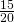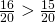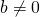Fraction

A fraction is a number representing a part of the whole e.g. ¼ (1 is the numerator & 4 is the denominator ) is showing whole 4 than ¼ is the 1/4th part.
Like fraction– fraction having the same denominator are called like fraction.
Unlike fraction– fractions having different denominator are called unlike fraction.
Proper fraction– a fraction whose numerator is less than the denominator is called proper fraction.e.g. 2/3
Improper fraction– a fraction whose numerator is greater than the denominator is called improper fraction.
Unit fraction– a fraction having numerator is 1 called a unit fraction ½, ¼,1/7 etc.
Mixed fraction– a fraction which is a combination of a whole number and a proper fraction is called a mixed fraction. E.g. 2 1/(3 )
Equivalent fraction– ½ =all these fractions being equal in value are called equivalent fractions.

Comparing Fractions
1. We find the LCM of their denominators.
2. We make the denominator of each fraction equal to the LCM & convert them into like fraction.
3. Then compare the numerator of fraction.and=andhere we can see thatAddition & subtraction of fraction– same to comparing procedure .
Fraction– a number of the form a/b where a & b are whole number &is called a fraction, a is called numerator b is the denominator
a fraction is the number representing a part of the whole.
Sum/difference of fraction = sum/difference of their numerator / common denominator